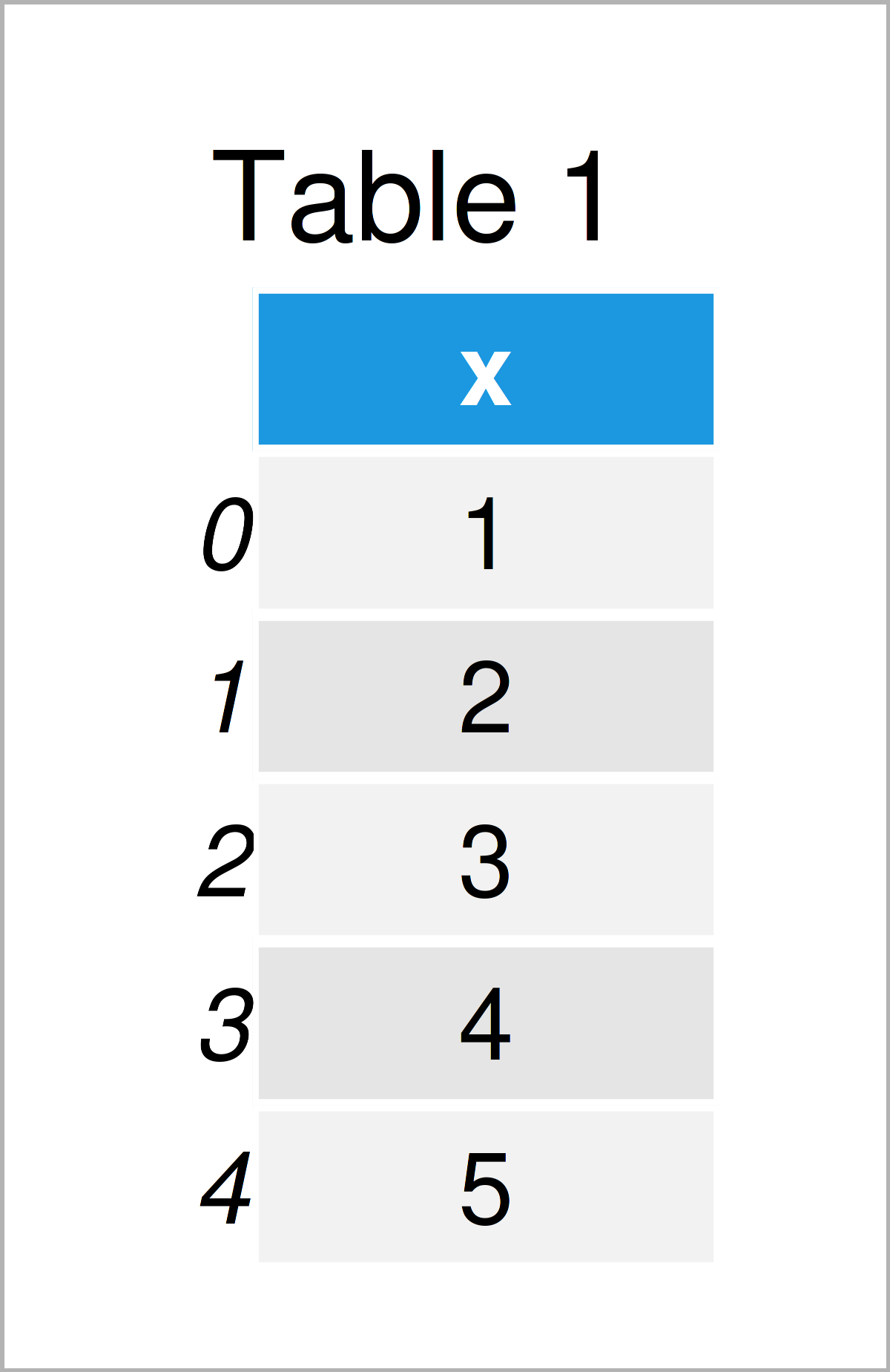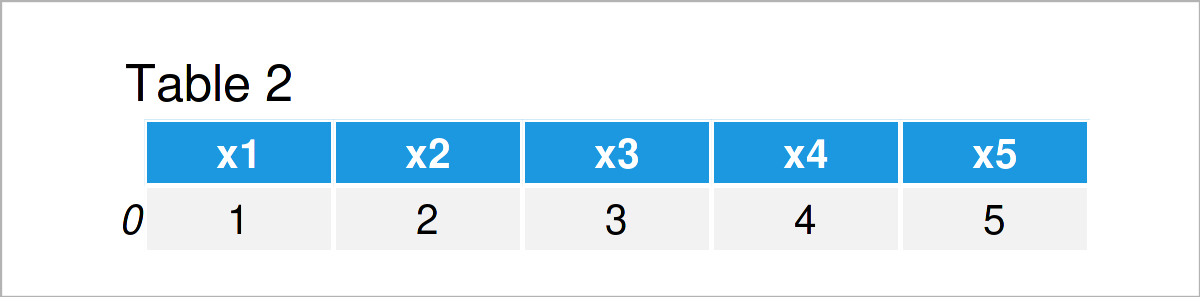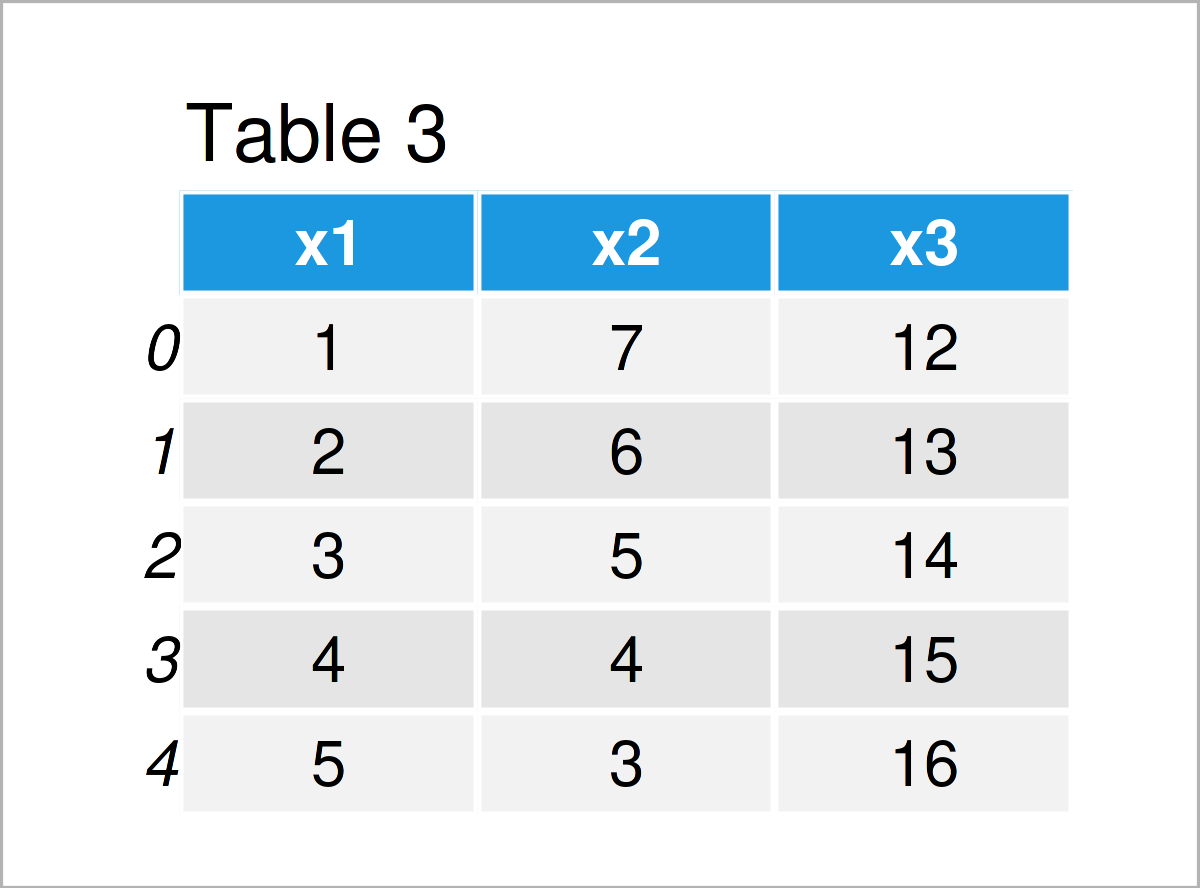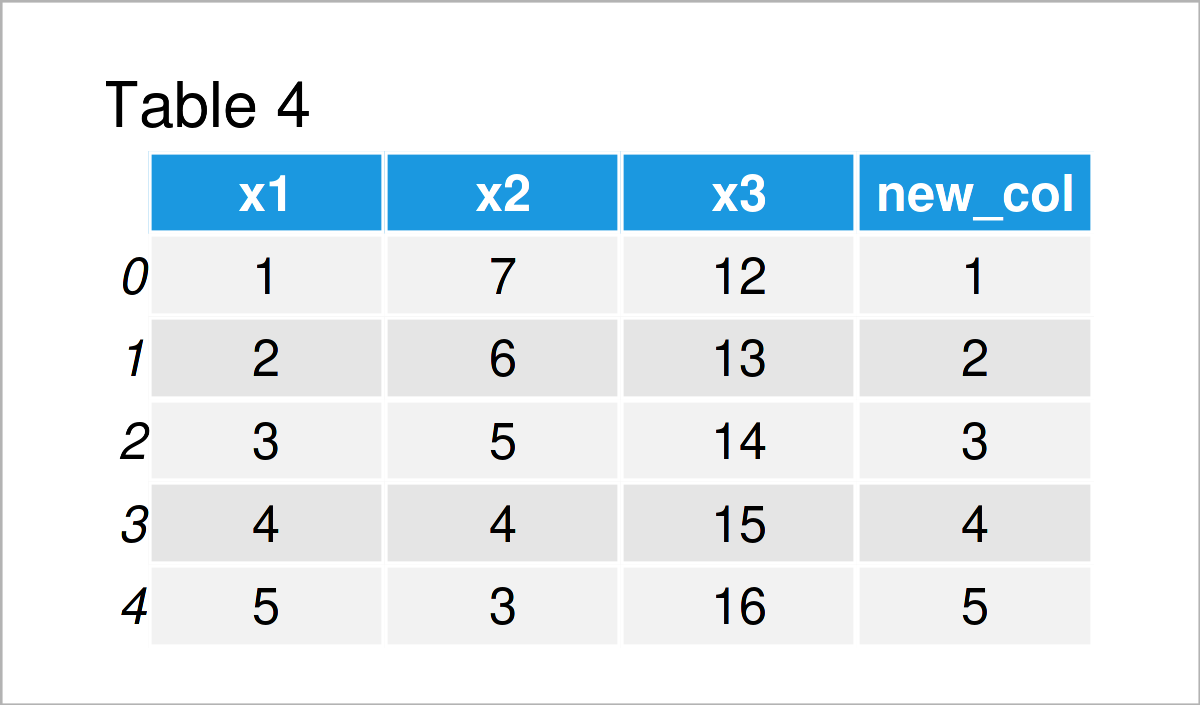# Convert List to pandas DataFrame in Python (3 Examples)

On this page you’ll learn how to create a pandas DataFrame from a list object in the Python programming language.

The page will consist of three examples for the creation of a pandas DataFrame from a list object. To be more precise, the content of the page looks as follows:

Let’s do this:

## Creating Example Data

We use the following list object as a basis for this Python tutorial:

```my_list = [1, 2, 3, 4, 5]                          # Create example list
print(my_list)                                     # Print example list
# [1, 2, 3, 4, 5]```

As you can see, our list contains five integer elements ranging from the values 1 to 5.

## Example 1: Convert List to pandas DataFrame Column

In Example 1, I’ll show how to construct a new pandas DataFrame based on a list using the Python programming language.

For the following tutorial, we’ll first need to import the pandas library:

`import pandas as pd                                # Import pandas library to Python`

In the next step, we can use the DataFrame function of the pandas library to convert our example list to a single column in a new pandas DataFrame:

```my_data1 = pd.DataFrame({'x': my_list})            # Create pandas DataFrame from list
print(my_data1)                                    # Print pandas DataFrame```Table 1 illustrates that our new pandas DataFrame is composed of five rows and one column. The values in this column correspond to the values in our list.

## Example 2: Convert Each List Element to Separate Column of pandas DataFrame

In this example, I’ll show how to create a pandas DataFrame with a new variable for each element in a list.

We can do this in two steps. First, we have to initialize our pandas DataFrame using the DataFrame function. Second, we have to set the column names of our DataFrame.

Consider the Python syntax below:

```my_data2 = pd.DataFrame([my_list])                 # Each list element as column
my_data2.columns = ['x1', 'x2', 'x3', 'x4', 'x5']  # Change column names
print(my_data2)                                    # Print pandas DataFrame```By running the previous syntax, we have created Table 2, i.e. another data set containing one row and a separate column for each element in our list.

## Example 3: Add List as New Column to Existing pandas DataFrame

This example explains how to append a list object as a new column to an already existing pandas DataFrame.

For this, we first have to create an exemplifying DataFrame:

```my_data3 = pd.DataFrame({'x1':range(1, 6),         # Create pandas DataFrame
'x2':range(7, 2, - 1),
'x3':range(12, 17)})
print(my_data3)                                    # Print pandas DataFrame```As shown in Table 3, we have created a new pandas DataFrame consisting of five rows and three columns.

Note that it’s important that the number of rows in this DataFrame are equal to the length of our list object.

In the next step, we can add our list object as a new variable to our pandas DataFrame:

```my_data3['new_col'] = my_list                      # Add list to existing DataFrame
print(my_data3)                                    # Print pandas DataFrame```As revealed in Table 4, the previous code has created an updated version of our input data set to which our list has been concatenated as a new variable.

## Video & Further Resources

Please accept YouTube cookies to play this video. By accepting you will be accessing content from YouTube, a service provided by an external third party.If you accept this notice, your choice will be saved and the page will refresh.

Besides that, you could have a look at some of the related articles on my website.

On this page you have learned how to convert a list to a pandas DataFrame in the Python programming language. In case you have any further questions or comments, please let me know in the comments.

Subscribe to the Statistics Globe Newsletter